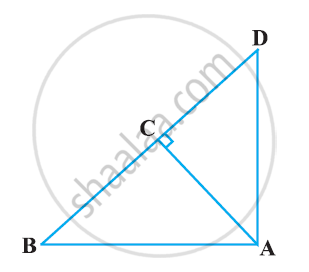# In Figure ABD is a triangle right angled at A and AC ⊥ BD. Show that AC^2 = BC × DC - Mathematics

In Figure ABD is a triangle right angled at A and AC ⊥ BD. Show that AC2 = BC × DC#### Solution

Let ∠CAB = x

In ΔCBA,

∠CBA = 180° - 90° - x

∠CBA = 90° - x

= 90° - x

∠CDA = 180° - 90° - (90° - x)

∠CDA = x

In ΔCBA and ΔCAD, we have

∠CAB = ∠CDA

∠ACB = ∠DCA (Each equals to 90°)

∴ ΔCBA ~ ΔCAD [By AAA similarity criterion]

⇒ (AC)/(DC) = (BC)/(AC)

⇒ AC2 = DC × BC

Concept: Right-angled Triangles and Pythagoras Property
Is there an error in this question or solution?

#### APPEARS IN

NCERT Class 10 Maths
Chapter 6 Triangles
Exercise 6.5 | Q 3.2 | Page 150